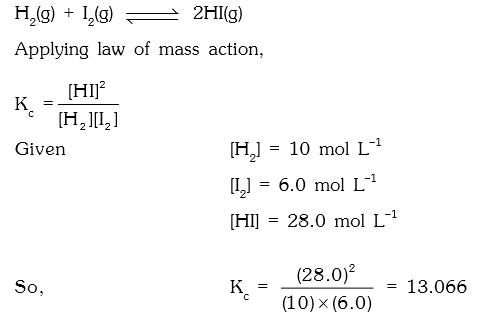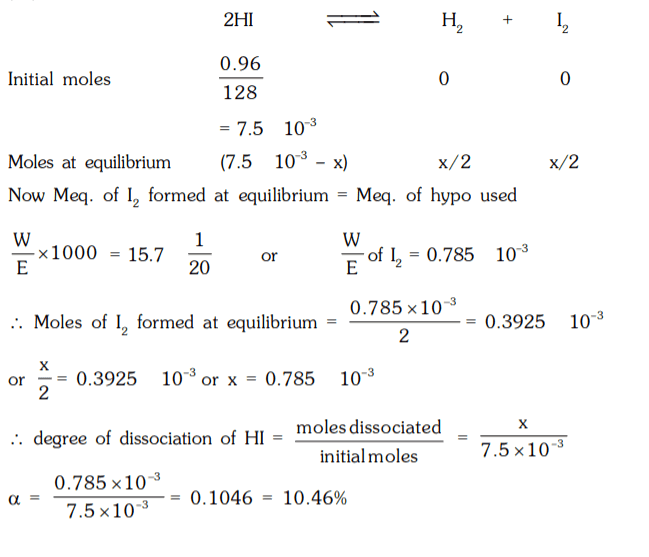## Chemical Equilibrium Questions and Answers Part-1

1. In the reaction, H2(g) + I2(g) ⇌ 2HI(g) the concentration of H2, I2 and HI at equilibrium are 10.0, 6.0 and 28 moles per litre respectively. What will be the equilibrium constant?
a) 13.066
b) 20.90
c) 29.40
d) 30.61

Explanation:2. The equilibrium constant for the reaction H2(g) + S (s) ⇌ H2S(g) ; is 18.5 at 925 K and 9.25 at 1000 K respectively. The enthalpy of the reaction will be :
a) –68000.05 J mol-1
b) –71080.57 J mol-1
c) –80071.75 J mol-1
d) 57080.75 J mol-1

Explanation:3. Chemical equilibrium is a condition :
a) where all species have concentration = 1
b) where all species have constant concentration with respect to time
c) where all species have same concentration
d) all of above

Explanation: Chemical equilibrium defined as when all species have constant concentration with respect to time.

4. Example of physical equilibria, is :
a) H2(g) + I2(g) ⇌ 2HI (g)
b) CaCO3 (s) ⇌ CaO (s)+ CO2(g)
c) H2O (s) ⇌ H2O (l)
d) PCl5(g) ⇌ PCl3 (g) + Cl2(g)

Explanation: Physical equilibria does not include any chemical change.

5. When ethanol and acetic acid were mixed together in equilimolecular proportion 66.6% are converted into ethyl acetate. Calculate Kc. Also calculate quantity of ester produced if one mole of acetic acid is treated with 0.5 mole and 4 mole of alcohol respectively.
a) 4, 0.93, 0.43
b) 0.93, 4, 0.43
c) 0.43, 0.93, 4
d) 4, 0.43, 0.93

Explanation:6. One mole of ammonium carbamate dissociate as shown below at 500 K.
NH2COONH4(s) ⇌ 2NH3(g) + CO2(g)
If the pressure exerted by the released gases is 6.0 atm, the value of KP is -
a) 32 atm
b) 8 atm
c) 3 atm
d) 7 atm

Explanation:7. The volume of a closed reaction vessel in which the equilibrium :
2SO2 (g) + O2(g) ⇌ 2SO3 (g) sets is halved, Now -
a) the rates of forward and backward reactions will remain the same.
b) the equilibrium will not shift.
c) the equilibrium will shift to the left.
d) the rate of forward reaction will become double that of reverse reaction and the equilibrium will shift to the right.

Explanation: In the reaction
2SO2 (g) + O2(g) ⇌ 2SO3(g)
In this reaction three moles (or volumes) of reactants are converted into two moles (or volumes) of products i.e. there is a decrease in volume and so if the volume of the reaction vessel is halved the equilibrium will be shifted to the right i.e. more product will be formed and the rate of forward reaction will increase i.e. double that of reverse reaction

8. H2(g) + I2(g) ⇌ 2HI (g)
When 92 g of I2 and 1g of H2 are heated at equilibrium at 450°C, the equilibrium mixture contained 1.9 g of I2. How many moles of I2 and HI are present at equilibrium.
a) 0.0052 & 0.347 moles
b) 0.0075 & 0.7094 moles
c) 0.0075 & 0.147 moles
d) 0.0050 & 0.147 moles

Explanation: moles of I2 taken = 92/254 = 0.3622
moles of H2 taken = 1/2 = 0.5
moles of I2 remaining = 1.9/254 = 0.0075
moles of I2 used = 0.3622 – 0.0075 = 0.3547
moles of H2 used = 0.3547
moles of H2 remaining = 0.5 – 0.3547 = 0.1453
moles of HI formed = 0.3547 × 2 = 0.7094
At equilibrium
moles of I2 = 0.0075 moles
moles of HI = 0.7094 moles

9. KP for the reaction N2 + 3H2 ⇌ 2NH3 at 400°C is 3.28 × 10-4. Calculate Kc
a) 0.4 mole-2 litre2
b) 0.6 mole-2 litre2
c) 1.0 mole-2 litre2
d) 0.3 mole-2 litre2

Explanation: N2 + 3H2 ⇌ 2NH3
∆n = –2 and KP = KC (RT)∆n
3.28 × 10–4 = Kc (0.0821 × 673)–2
and Kc = 1.0 mole-2 litre2

10. 0.96 g of HI were heated to attain equilibrium 2 HI ⇌ H2 + I2. The reaction mixture on titration requires 15.7 mL of N/20 hypo. Calculate % dissociation of HI.
a) 21.9%
b) 19.9%
c) 18.9%
d) 10.46%